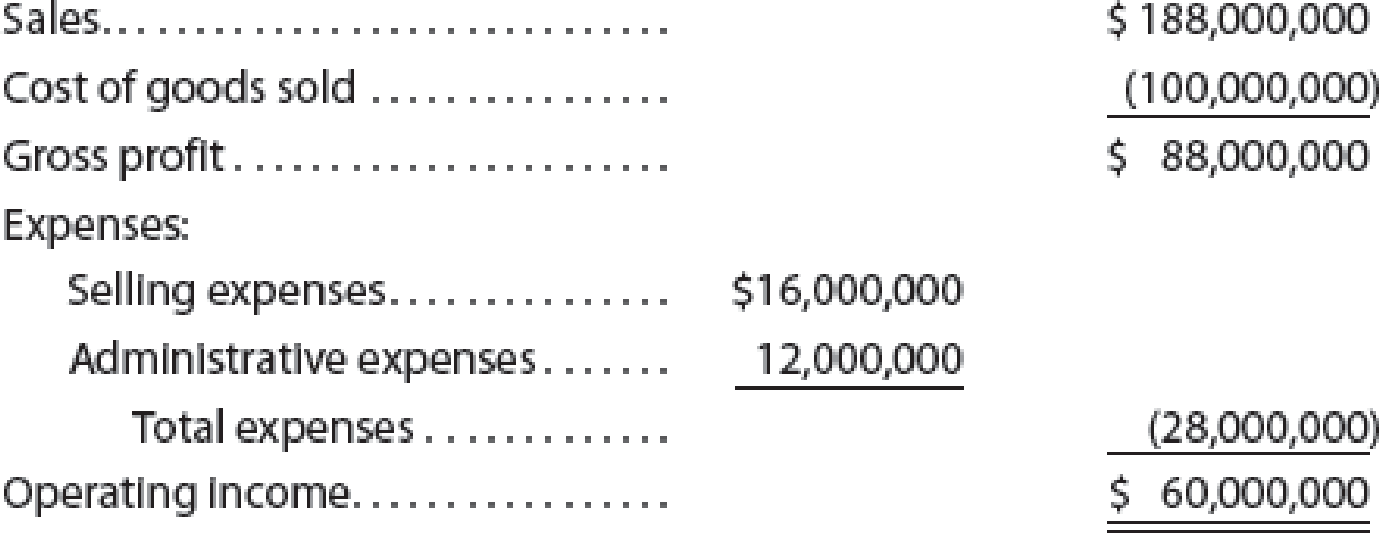Chapter 20, Problem 2PA

Chapter
Section
Textbook Problem

Break-even sales under present and proposed conditionsPortmann Company, operating at full capacity, sold 1,000,000 units at a price of $188 per unit during the current year. Its income statement is as follows:The division of costs between variable and fixed is as follows:Management is considering a plant expansion program for the following year that will permit an increase of$11,280,000 in yearly sales. The expansion will increase fixed costs by $5,000,000 but will not affect the relationship between sales and variable costs.Instructions 1. Determine the total variable costs and the total fixed costs for the current year. 2. Determine (a) the unit variable cost and (b) the unit contribution margin for the current year. 3. Compute the break-even sales (units) for the current year. 4. Compute the break-even sales (units) under the proposed program for the following year. 5. Determine the amount of sales (units) that would be necessary under the proposed program to realize the$60,000,000 of operating income that was earned in the current year. 6. Determine the maximum operating income possible with the expanded plant. 7. If the proposal is accepted and sales remain at the current level, what will the operating income or loss be for the following year? 8. Based on the data given, would you recommend accepting the proposal? Explain.

1.

To determine

Calculate the total fixed costs and the total variable costs for the current year.

Explanation

Variable costs: These are the costs that proportionately change with the changes in the activity base such as units of production.

Fixed Costs: These are the costs that remain constant in total dollar amount irrespective to the changes in the activity base such as units of production.

Determine the total variable cost.

 Particulars Total cost (A) Variable cost percentage (B) Variable cost (A×B) Cost of Goods sold $100,000,000 70%$70,000,000 Selling expenses $16,000,000 75%$12,000,000 Administrative expenses $12,000,000 50%$6,000,000

2(a)

To determine

Calculate the unit variable cost for the current year.

2(b)

To determine

Calculate the unit contribution margin for the current year.

3.

To determine

Compute the break-even sales (units) for the current year.

4.

To determine

Compute the break-even sales (units) under the proposed program for the following year.

5.

To determine

Calculate the amount of sales (units) if the company desires a target profit of \$60,000,000.

6.

To determine

Calculate the maximum operating income possible with the expanded plant.

7.

To determine

Calculate the operating income or loss for the following year, if the proposal is accepted and the sales remains same.

8.

To determine

Explain whether to recommend for accepting the proposal.

Still sussing out bartleby?

Check out a sample textbook solution.

See a sample solution

The Solution to Your Study Problems

Bartleby provides explanations to thousands of textbook problems written by our experts, many with advanced degrees!

Get Started

Why is productivity important?

Brief Principles of Macroeconomics (MindTap Course List)

Why is the American economy called a mixed economy?

Foundations of Business (MindTap Course List)

Discuss the similarities and differences between the discounted dividend and corporate valuation models.

Fundamentals of Financial Management, Concise Edition (with Thomson ONE - Business School Edition, 1 term (6 months) Printed Access Card) (MindTap Course List)